# Machine Learning Tutorials

Learn how to build machine learning and deep learning models for many purposes in Python using popular frameworks such as TensorFlow, PyTorch, Keras and OpenCV.##### How to Fine Tune ViT for Image Classification using Transformers in Python

Learn how to fine tune the Vision Transformer (ViT) model for the image classification task using the Huggingface Transformers, evaluate, and datasets libraries in Python.##### How to Perform Image Segmentation using Transformers in Python

Learn how to use image segmentation transformer model to segment any image using huggingface transformers and PyTorch libraries in Python.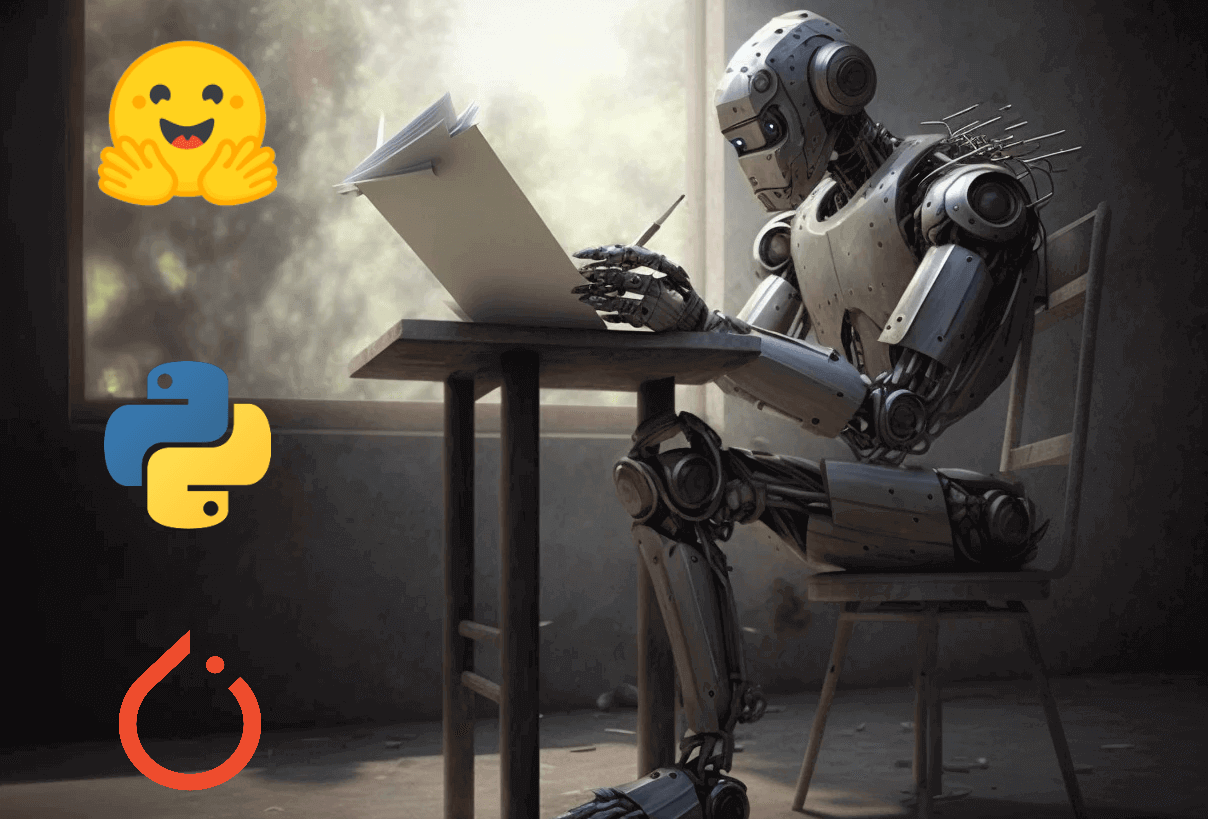##### Image Captioning using PyTorch and Transformers in Python

Learn how to use pre-trained image captioning transformer models and what are the metrics used to compare models, you'll also learn how to train your own image captioning model with Pytorch and transformers in Python.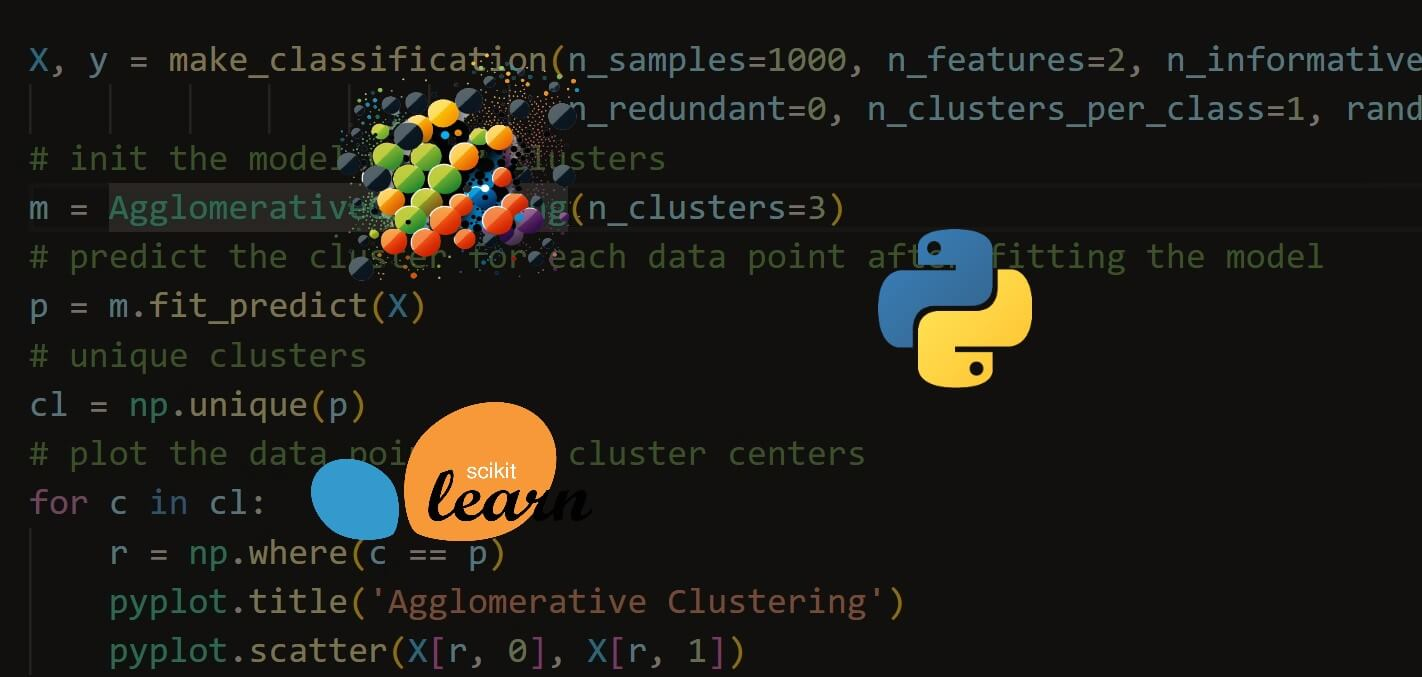##### Clustering Algorithms in Machine Learning with Python

This article is a must-read for anyone looking to unlock the full potential of clustering in machine learning! It delves into the world of clustering, exploring different types such as density-based and centroid-based, and introducing lesser-known techniques like hierarchical and monothetic clustering with Python.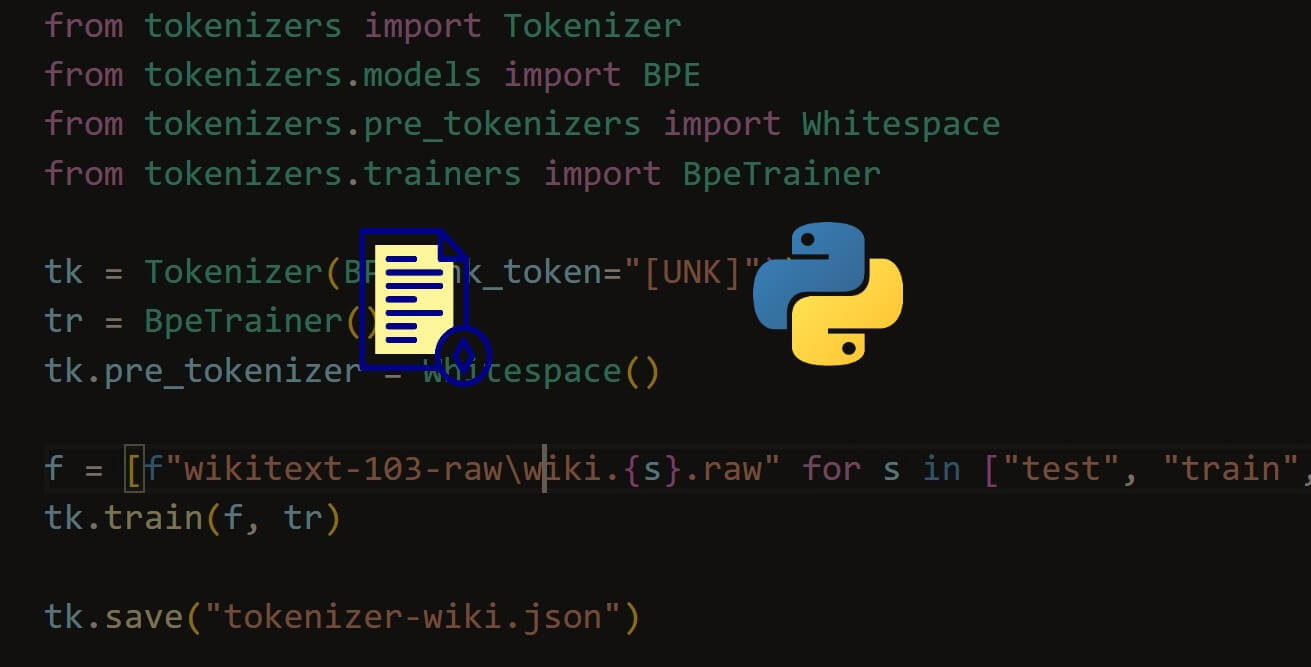##### Tokenization, Stemming, and Lemmatization in Python

This article discusses the preprocessing steps of tokenization, stemming, and lemmatization in natural language processing. It explains the importance of formatting raw text data and provides examples of code in Python for each procedure.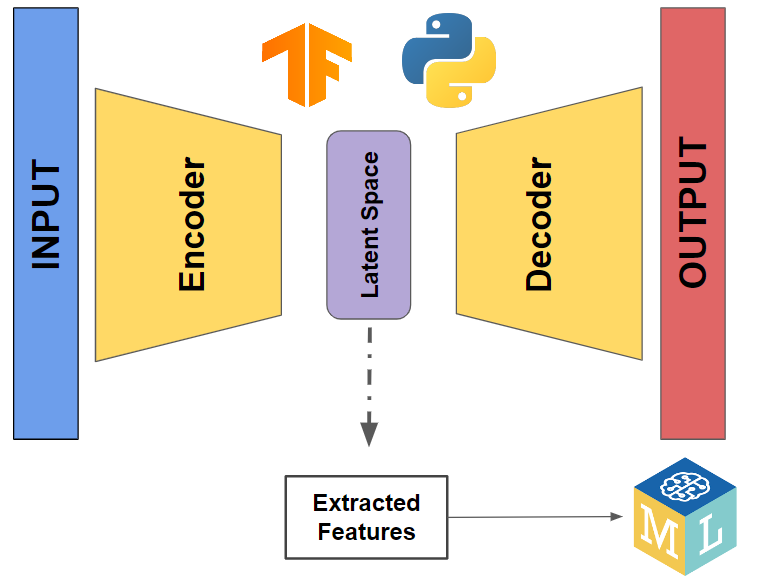##### Autoencoders for Dimensionality Reduction using TensorFlow in Python

Learn how to benefit from the encoding/decoding process of an autoencoder to extract features and also apply dimensionality reduction using Python and Keras all that by exploring the hidden values of the latent space.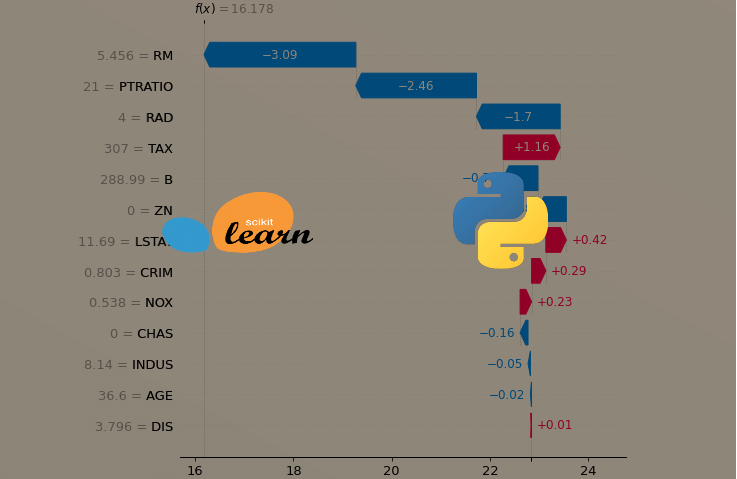##### A Guide to Explainable AI Using Python

An overview of model explainability and interpretability fundamentals, AI applications, and biases in AI model predictions. We looked at utilizing SHAP and LIME to explain a Logistic Regression model and how to explain and interpret an ensemble model.##### Dimensionality Reduction Using Feature Selection in Python

Learn how to perform dimensionality reduction with feature selection such as recursively eliminating features, handling highly correlated features, and more using Scikit-learn in Python.##### Dimensionality Reduction: Feature Extraction using Scikit-learn in Python

Learn how to perform different dimensionality reduction using feature extraction methods such as PCA, KernelPCA, Truncated SVD, and more using Scikit-learn library in Python.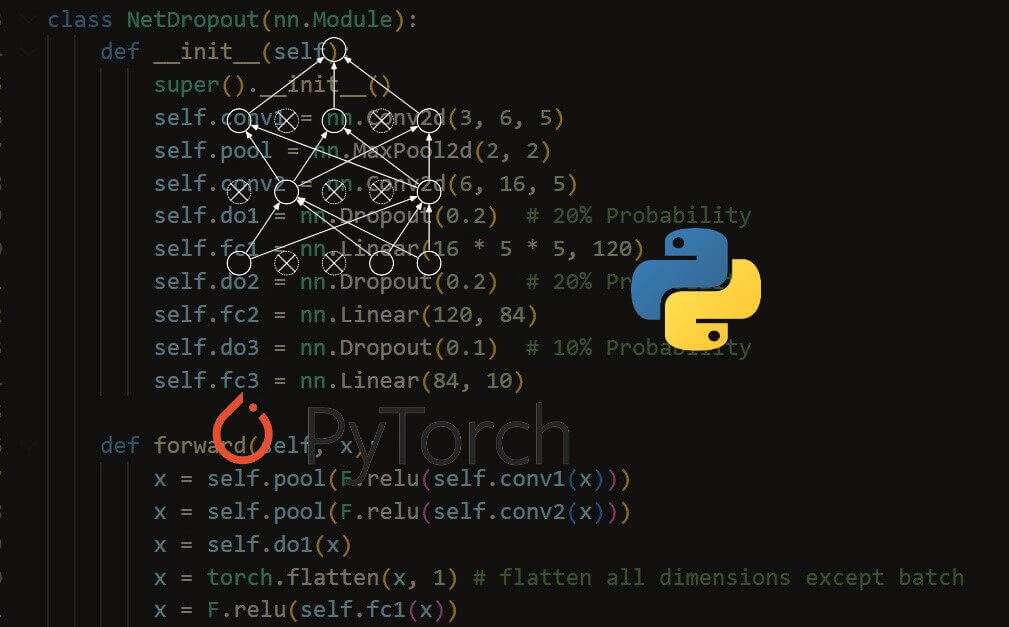##### Dropout Regularization using PyTorch in Python

Learn the importance of dropout regularization and how to apply it in PyTorch Deep learning framework in Python.##### Logistic Regression using PyTorch in Python

Learn how to perform logistic regression algorithm using the PyTorch deep learning framework on a customer churn example dataset in Python.##### Handling Imbalanced Datasets: A Case Study with Customer Churn

Learn how to handle one of the main data science common problems, which are imbalanced datasets, how to deal with them using SMOTE, tweaking class weights, and resampling in Python.